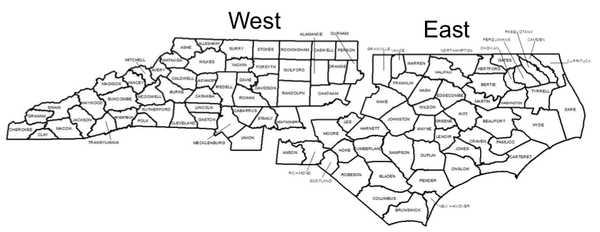NC State Extension Publications

## Introduction

Many forest landowners have good reasons to be interested in historic North Carolina timber stumpage (standing timber) prices. Not only is such information useful in assessing current market opportunities and analyzing forest management options, but it may be the best or only way to calculate a landowner’s basis (for income tax purposes) in timber to be sold. These historic price data have been compiled from various sources (primarily Timber Mart-South since 1976). Annual market prices are averages of stumpage prices reported at the time. Considerable additional information on these products and others, as well as other states and regions, are available from the collectors and distributors of price information.

Stumpage price data are reported for four timber product categories (pine sawtimber and pulpwood, and mixed hardwood sawtimber and pulpwood) for western North Carolina, eastern North Carolina, statewide, and southeast, United States. Eastern North Carolina is defined as the region east of, and including, Granville, Wake, Lee, Moore, Richmond and Anson counties (Figure 1). Western North Carolina is defined as the region west of and including Person, Durham, Chatham, Montgomery, Stanley, and Union counties (Figure 1).Figure 1: Map of North Carolina counties divided into two regions, east and west.

Created by Jessica Knight

 Year Eastern NC Western NC State-wide Average Southeast-wide Average 1976 $135.00$38.00 $86.00 1977$131.00 $56.00$91.00 1978 $127.00$73.00 $103.00 1979$160.00 $89.00$147.00 1980 $131.00$78.00 $120.00 1981$175.00 $84.00$153.00 1982 $148.00$67.00 $134.00 1983$178.00 $83.00$155.00 1984 $168.00$78.00 $155.00 1985$149.00 $75.00$132.00 1986 $157.00$90.00 $141.00 1987$142.00 $81.00$117.00 1988 $155.00$78.00 $121.00 1989$169.00 $79.00$133.00 1990 $185.00$84.00 $140.00 1991$182.00 $90.00$133.00 $127.00 1992$193.00 $95.00$144.00 $160.00 1993$187.00 $108.00$147.00 $189.00 1994$222.00 $134.00$178.00 $248.00 1995$231.00 $110.00$170.00 $268.00 1996$262.00 $98.00$180.00 $237.00 1997$267.00 $146.00$207.00 $291.00 1998$309.00 $165.00$237.00 $304.00 1999$329.00 $194.00$261.00 $290.23 2000$345.00 $220.00$282.00 $293.36 2001$332.00 $215.00$274.00 $261.62 2002$317.00 $238.00$277.00 $273.64 2003$292.00 $249.00$271.00 $270.53 2004$286.00 $188.00$237.00 $285.54 2005$299.00 $221.00$260.00 $300.21 2006$309.00 $216.00$262.00 $286.86 2007$297.00 $202.00$249.00 $277.86 2008$262.00 $217.00$239.00 $233.10 2009$220.00 $174.00$197.00 $202.00 2010$247.00 $210.00$229.00 $210.00 2011$209.00 $153.00$181.00 $180.00 2012$203.00 $163.00$183.00 $175.00 2013$217.00 $184.00$201.00 $185.00 2014$212.00 $185.00$198.00 $192.00 2015$201.00 $169.00$185.00 $193.00 2016$221.15 $160.41$190.78 $186.00 2017$204.57 $155.56$180.07 $177.25 2018$211.22 $166.35$188.80 $179.25 2019$253.80 $172.71$213.25 $182.78 2020$206.17 $153.98$180.08 $164.00 2021$248.08 $188.82$218.45 $193.16 2022$255.94 $192.41$224.21 $201.88 ## Pine Pulpwood Stumpage Price History ($/Cord, Scribner log rule)

Skip to Pine Pulpwood Stumpage Price History ($/Cord, Scribner log rule)  Year Eastern NC Western NC State-wide Average Southeast-wide Average 1976$6.00 $5.50$6.00 1977 $7.85$5.30 $6.75 1978$8.85 $5.40$7.25 1979 $8.20$6.15 $7.65 1980$8.50 $6.05$7.75 1981 $9.30$5.90 $8.25 1982$9.40 $5.90$8.75 1983 $10.35$6.15 $9.25 1984$11.00 $6.70$10.55 1985 $11.00$6.85 $9.90 1986$9.65 $6.55$8.60 1987 $11.20$10.10 $10.75 1988$12.54 $10.98$11.72 1989 $11.91$10.77 $11.39 1990$13.06 $11.80$12.79 1991 $16.50$14.88 $15.71$17.17 1992 $15.88$15.54 $15.71$19.67 1993 $17.21$18.03 $17.62$22.24 1994 $15.65$16.93 $16.29$22.73 1995 $15.16$16.92 $16.04$23.49 1996 $13.51$13.98 $13.74$23.73 1997 $14.42$14.42 $14.42$26.92 1998 $18.42$15.63 $17.02$28.54 1999 $18.08$14.96 $16.52$24.31 2000 $19.56$15.44 $17.50$20.86 2001 $17.25$13.24 $15.25$17.33 2002 $15.25$11.84 $13.54$16.24 2003 $17.62$14.11 $15.86$18.37 2004 $16.94$11.69 $14.31$17.50 2005 $17.46$16.25 $16.85$19.64 2006 $17.25$13.17 $15.21$17.58 2007 $18.25$12.26 $15.26$21.05 2008 $18.42$13.79 $16.11$23.16 2009 $18.94$14.03 $16.49$21.82 2010 $23.90$19.82 $21.86$25.58 2011 $19.10$16.38 $17.74$21.87 2012 $20.53$15.17 $17.85$23.50 2013 $24.81$17.59 $21.20$25.94 2014 $24.09$17.01 $20.55$27.72 2015 $25.69$16.68 $21.19$27.16 2016 $34.44$18.91 $26.68$27.26 2017 $36.71$21.78 $29.25$25.27 2018 $36.70$21.51 $29.12$24.56 2019 $28.47$21.55 $25.01$24.64 2020 $30.06$18.56 $24.31$22.62 2021 $34.63$21.11 $27.87$25.82 2022 $30.11$19.87 $24.99$27.21

 Year Eastern NC Western NC State-wide Average Southeast-wide Average 1976 $50.00$30.00 $40.00 1977$53.00 $38.00$45.00 1978 $55.00$48.00 $50.00 1979$53.00 $47.00$49.00 1980 $49.00$41.00 $46.00 1981$53.00 $46.00$53.00 1982 $51.00$55.00 $50.00 1983$52.00 $57.00$54.00 1984 $43.00$52.00 $47.00 1985$43.00 $53.00$49.00 1986 $49.00$61.00 $54.00 1987$62.00 $79.00$67.00 1988 $61.00$81.00 $73.00 1989$84.00 $88.00$84.00 1990 $65.00$94.00 $81.00 1991$77.00 $86.00$87.00 $123.00 1992$88.00 $98.00$93.00 $94.00 1993$118.00 $114.00$116.00 $134.00 1994$105.00 $108.00$107.00 $124.00 1995$114.00 $126.00$120.00 $141.00 1996$130.00 $109.00$120.00 $124.00 1997$152.00 $129.00$141.00 $142.00 1998$168.00 $148.00$158.00 $153.00 1999$146.00 $157.00$152.00 $149.03 2000$176.00 $163.00$170.00 $153.21 2001$202.00 $205.00$203.00 $161.88 2002$194.00 $204.00$199.00 $165.20 2003$206.00 $206.00$206.00 $175.72 2004$203.00 $167.00$185.00 $181.76 2005$199.00 $188.00$193.00 $189.46 2006$221.00 $205.00$213.00 $182.72 2007$195.00 $164.00$179.00 $192.00 2008$168.00 $176.00$172.00 $193.00 2009$157.00 $138.00$147.00 $179.00 2010$190.00 $162.00$176.00 $202.00 2011$177.00 $162.00$170.00 $190.00 2012$175.00 $155.00$165.00 $196.00 2013$232.00 $199.00$216.00 $219.00 2014$242.00 $220.00$231.00 $265.00 2015$249.00 $193.00$221.00 $275.00 2016$241.45 $173.14$207.29 $287.25 2017$228.90 $209.41$219.16 $270.00 2018$236.86 $204.93$215.88 $275.35 2019$232.61 $215.11$223.86 $278.95 2020$211.34 $190.92$201.13 $275.75 2021$238.53 $243.77$241.15 $274.91 2022$267.11 $270.96$269.04 $292.54 ## Hardwood Pulpwood Stumpage Price History ($/Cord, Doyle log rule)

Skip to Hardwood Pulpwood Stumpage Price History ($/Cord, Doyle log rule)  Year Eastern NC Western NC State-wide Average Southeast-wide Average 1976$2.50 $2.75$2.71 1977 $2.85$3.10 $3.00 1978$3.00 $3.10$3.05 1979 $2.50$3.00 $2.75 1980$3.05 $3.20$3.00 1981 $3.35$3.20 $3.25 1982$3.00 $3.90$3.70 1983 $2.65$3.75 $3.40 1984$2.00 $5.00$3.75 1985 $2.00$4.95 $3.35 1986$2.00 $4.05$2.75 1987 $3.90$6.20 $5.15 1988$3.79 $6.04$4.80 1989 $4.21$7.68 $6.32 1990$5.00 $8.00$6.75 1991 $6.00$8.88 $7.74$6.52 1992 $8.31$10.40 $9.35$8.58 1993 $12.32$12.58 $12.45$10.86 1994 $8.18$11.70 $9.94$10.88 1995 $10.39$12.53 $11.46$15.46 1996 $7.91$13.00 $10.46$13.73 1997 $8.02$12.20 $10.11$16.55 1998 $8.94$13.10 $11.02$16.08 1999 $7.96$11.92 $9.94$12.38 2000 $9.26$13.36 $11.31$12.01 2001 $8.87$11.39 $10.13$14.52 2002 $7.70$13.79 $10.74$14.49 2003 $13.18$16.72 $14.95$20.24 2004 $8.63$15.42 $12.03$17.15 2005 $9.43$13.24 $11.33$19.73 2006 $8.98$12.13 $10.55$16.94 2007 $12.83$16.12 $14.48$19.23 2008 $15.18$16.22 $15.70$21.68 2009 $14.43$13.94 $14.19$22.49 2010 $16.30$17.51 $16.90$27.28 2011 $13.43$16.89 $15.16$19.56 2012 $10.43$12.29 $11.36$21.48 2013 $13.82$14.23 $14.02$25.46 2014 $14.92$16.78 $15.85$31.01 2015 $17.92$18.05 $17.99$26.41 2016 $12.93$16.54 $14.74$26.77 2017 $9.54$14.12 $11.83$22.85 2018 $7.86$15.21 $11.54$26.90 2019 $14.08$18.17 $16.13$31.94 2020 $16.17$13.43 $14.80$23.58 2021 $22.04$19.72 $20.88$27.07 2022 $22.29$19.80 $21.04$32.14

# Author

Associate Dean of Extension & Professor
Forestry & Environmental Resources